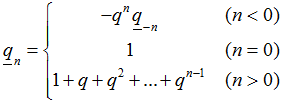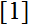# Volume 10, 2004, Number 2

Volume 10Number 1 ▷ Number 2 ▷ Number 3Number 4

Some properties of Fermatian numbers
Original research paper. Pages 25—33
A. G. Shannon
Full paper (152 Kb) | Abstract

This paper looks at some basic number theoretic properties of Fermatian numbers. We define the n-th reduced Fermatian number in terms ofso that 1n = n, and 1n! = n!, where qn! = qnqn−1q1.
Some congruence properties and relationships with Bernoulli and Fibonacci numbers are explored. Some aspects of the notation and meaning of the Fermatian numbers are also outlined.

On five Smarandache’s problems
Original research paper. Pages 34—53
Mladen V. Vassilev-Missana and Krassimir T. Atanassov
Full paper (5956 Kb)

Some representations concerning the product of divisors of n
Original research paper. Pages 54—56
Full paper (896 Kb) | Abstract

Let us denote by τ(n) the number of all divisors of n. It is well-known (see, e.g.,) that(1)

and of course, we have(2)

But (1) is not a good formula for Pd(n), because it depends on function τ and to express τ(n) we need the prime number factorization of n.
Below, we give other representations of Pd(n) and pd(n), which do not use the prime number factorization of n.

Volume 10Number 1 ▷ Number 2 ▷ Number 3Number 4